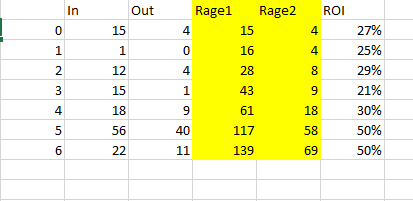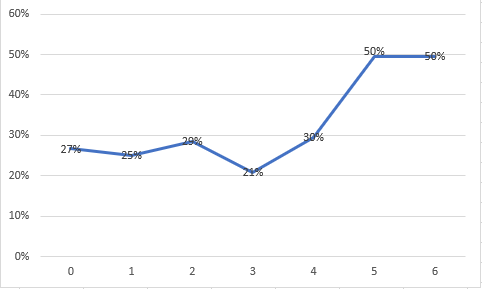# App Development

Discussion board where members can learn more about Qlik Sense App Development and Usage.

Announcements
Welcome to Qlik Community! Check out our new navigation! FIND OUT MORE
cancel
Showing results for
Did you mean:Partner - Contributor III

## Cumulative total for relative value

Hello, everybody!

Im try to calculate a relative value for chart visualisation where dimantion in days spend (0,1,2 etc)

Problem: in component of formula i need a cumulative total by my dimantion.

I'm make some example:Im wont get chart like thisthe problem is that I cannot use the previous values ​​for the calculation. I need to take a measurement and count less than the current in a variable or in SetAnalysis

Labels (3)

• ### total sum by dimantion

1 Solution

Accepted SolutionsMVP

may be this

``=(rangesum(above(total sum({<ID>}OUT),0,RowNo(total)))/rangesum(above(total sum({<ID>}IN),0,RowNo(total))))*avg(1)``

Where ID is the X-axis Dimension

2 RepliesMVP

may be this

``=(rangesum(above(total sum({<ID>}OUT),0,RowNo(total)))/rangesum(above(total sum({<ID>}IN),0,RowNo(total))))*avg(1)``

Where ID is the X-axis DimensionPartner - Contributor III
Author

Thenks, its work.

Im make a reference table and add it to model with references to main table. Im need it becose my formula with count(distinct id) ... so im need to calculate unicu id in each row...Tags
Community Browser